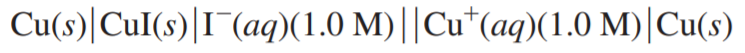×
Get Full Access to Chemistry: A Molecular Approach - 3 Edition - Chapter 18 - Problem 117e
Get Full Access to Chemistry: A Molecular Approach - 3 Edition - Chapter 18 - Problem 117e

×

# The Ksp of CuI is 1.1 × 10-12. Find Ecell for the cell:ISBN: 9780321809247 1

## Solution for problem 117E Chapter 18

Chemistry: A Molecular Approach | 3rd Edition

• Textbook Solutions
• 2901 Step-by-step solutions solved by professors and subject experts
• Get 24/7 help from StudySoup virtual teaching assistantsChemistry: A Molecular Approach | 3rd Edition

4 5 1 353 Reviews
23
0
Problem 117E

The Ksp of CuI is 1.1 x 10-12. Findfor the cell:Step-by-Step Solution:
Step 1 of 3

Chapter 3 Notes >Vocabulary Actual Yield: the amount of product that is actually obtained Atomic Mass: the weighted average of the masses of all the isotopes of that element Combination: two or more reactants form one product Combustion: reactions that involve oxygen molecules and produce energy Decomposition: one reactant falls apart into two or more products. Empirical Formula: smallest whole number ratio of atoms present in a compound Law of Conservation of Mass: Mass can neither be created nor destroyed Limiting Reactant: the reactant that is entirely consumed when a reaction goes to completion Molar Mass: is the mass in grams of one mole of the substance Mole: (6.022 x 10 ) is the number equal to the number of carbon atoms in 12.01 grams of carbon Molecular Formula: describe the type and number of atoms in a molecule Percent Composition: describes the percentage by mass of each element in a compound Percentage Yield: a comparison of actual to theoretical yield Theoretical Yield: the maximum amount of product that can be obtained by a reaction from given amounts of reactants >Concepts Chemical Reactions •Converts one substance to another •Law of conservation of mass •Breaking bonds & Forming new bonds •Combination and Decomposition •Clues that a chemical rxn has occurred: - Color change - Precipitate forms - Bubbles form - Flame is produced Balancing Equations •Subscripts can never be changed •Coeﬃcients used to balance •A balanced equation has the same number of atoms of each element on

Step 2 of 3

Step 3 of 3

##### ISBN: 9780321809247

Unlock Textbook Solution